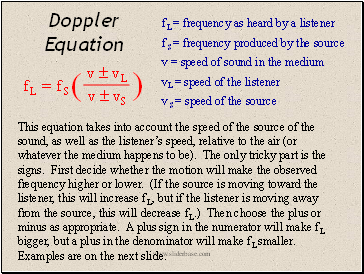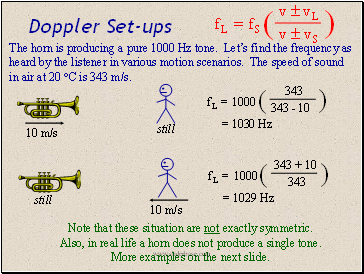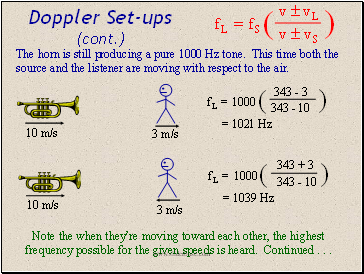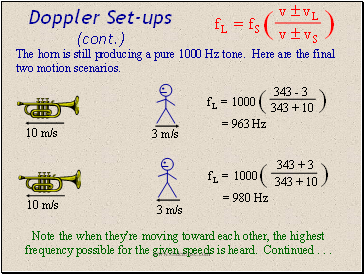# SoundPage 6

#### WATCH ALL SLIDES

When a source of sound is moving at the speed of sound, the wavefronts pile up on top of each other. This makes their combined amplitude very large, resulting in a shock wave and a sonic boom. At supersonic speeds a “Mach cone” is formed. The faster the source compared to sound, the smaller the shock wave angle will be.

Slide 21Doppler Equation

f L = frequency as heard by a listener

f S = frequency produced by the source

v = speed of sound in the medium

vL = speed of the listener

v S = speed of the source

This equation takes into account the speed of the source of the sound, as well as the listener’s speed, relative to the air (or whatever the medium happens to be). The only tricky part is the signs. First decide whether the motion will make the observed frequency higher or lower. (If the source is moving toward the listener, this will increase f L, but if the listener is moving away from the source, this will decrease f L.) Then choose the plus or minus as appropriate. A plus sign in the numerator will make f L bigger, but a plus in the denominator will make f L smaller. Examples are on the next slide.

Slide 22Doppler Set-ups

still

10 m/s

f L = 1000

343

343 - 10

)

(

= 1030 Hz

The horn is producing a pure 1000 Hz tone. Let’s find the frequency as heard by the listener in various motion scenarios. The speed of sound in air at 20 C is 343 m/s.

still

10 m/s

f L = 1000

343 + 10

343

)

(

= 1029 Hz

Note that these situation are not exactly symmetric. Also, in real life a horn does not produce a single tone. More examples on the next slide.

Slide 233 m/s

Doppler Set-ups (cont.)

10 m/s

f L = 1000

343 - 3

343 - 10

)

(

= 1021 Hz

The horn is still producing a pure 1000 Hz tone. This time both the source and the listener are moving with respect to the air.

f L = 1000

343 + 3

343 - 10

)

(

= 1039 Hz

10 m/s

3 m/s

Note the when they’re moving toward each other, the highest frequency possible for the given speeds is heard. Continued . . .

Slide 2410 m/s

10 m/s

3 m/s

Doppler Set-ups (cont.)

f L = 1000

343 - 3

343 + 10

)

(

= 963 Hz

The horn is still producing a pure 1000 Hz tone. Here are the final two motion scenarios.

f L = 1000

343 + 3

343 + 10

)

(

= 980 Hz

3 m/s

Note the when they’re moving toward each other, the highest frequency possible for the given speeds is heard. Continued . . .

Slide 25## Doppler Problem

Go to page:
1  2  3  4  5  6  7  8  9  10  11  12  13